Example Questions

Example Question #4 : How To Multiply Integers

George is buying his first car. He has $500 for a downpayment and can afford monthly payments of$175 for three years. What is the most expensive car he can purchase?

$4,750$8,000

$5,250$7.500

$6,800 Correct answer:$6,800

Explanation:

The most expensive car he can purchase is given by the total of all monthly payments and the down payment: $175 per month * 12 months per year * 3 years +$500 down = \$6,800

Example Question #5 : How To Multiply Integers

Given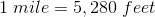, convert 60 miles per hour to approximate feet per second.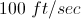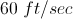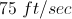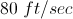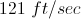Explanation:

Using the factor-label method we get: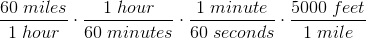approximately equals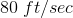The units are treated like numbers and are cancelled when they are in both the  numerator and denominator.

Example Question #6 : How To Multiply Integers

Which of the following expressions must be an even integer if x is an integer?

x6

3x

x + 3

x/8

x6

Explanation:

An integer raised to an even power always yields an even integer. All of the other expressions could yield odd integers.

Example Question #7 : How To Multiply Integers

Each variable f, g, h, i, and j represents a positive real number. Considering the equations below, which of the four variables g, h, i, or j necesarily has the greatest value?

1.75g = f

1.69h = f

1.23i = f

0.46j = f

h

g

i

j

j

Explanation:

All four variables are positive real numbers. Each variable g, h, i, and j are multiplied by differing values to obtain the same value f. Since j is multiplied by the smallest value to obtain f it must be the greatest value. In this manner we could determine that g is necesarily the smallest value.

Example Question #8 : How To Multiply Integers

If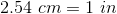, approximately how many cm are in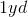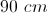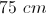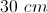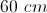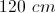Explanation:

Use the factor-label method for conversion: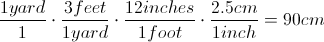The units are considered like numbers and can be cancelled out of both the numerator and the denominator.

Example Question #9 : How To Multiply Integers

Jordan is packing his lunch, and has decided to bring one piece of fruit, one vegetable, one type of sandwich, and one drink. How many different combinations of lunch can Jordan pack?

 Fruit Vegetable Sandwich Drink Apple Carrot Turkey Milk Banana Celery Chicken Water Orange Veggie

14

10

4

36

12

36

Explanation:

To find the number of different combinations, we must use the fundamental counting principal to multiply the number of options in each category together:

(3)(2)(3)(2) = 36

Example Question #1 : How To Add Integers

Solve the following equation.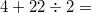Explanation:

This question is testing the order of operations. For the equation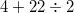, you must do the division problem first, then addition.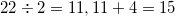.

Example Question #2 : How To Add Integers

A functionis defined as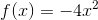. What is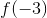?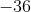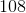Explanation:

To answer this question, we must substitute our value ofinto our equation forand follow our order of operations rules to solve for.

we first must substitute our known value into the equation. For this data: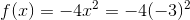We must now follow our order of operations to determine what we should do next. The order of operations goes as follows: parentheses, exponents, multiplication and division, and finally, addition and subtraction. So, for this equation, we must square thebecause solving for exponents comes before solving multiplication.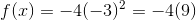Note that when you square a number, you are multiplying it by itself. When we multiply two negative numbers, their product is positive.

We then multiply our two integers together and solve for: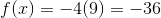Therefore, the answer to our question is.

Example Question #3 : How To Add Integers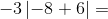Explanation:

To solve this question, we need to understand how absolute value works within an equation.

When numbers are within an absolute value sign (the numbers will be surrounded by), we need to use absolute value, or how far the number inside is away from zero. We must do any operations within the absolute value sign first. Therefore, the answer inside will always be positive, despite its sign, because you can't have negative distance from zero. So, for this data: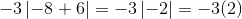We can then just multiply our two integers in order to get our answer: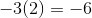Therefore, our answer is.

Example Question #4 : How To Add Integers

If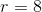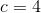, and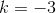, what does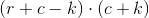equal?Explanation:

To answer this question, we must substitute our given values into an equation and solve, remembering our order of operations.

First, we must take our values for each variable and substitute them into the equation given. Therefore, since we are told that, and,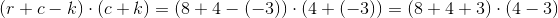Now that we have our values plugged in, we need to remember the order of operations in order to solve this problem. The order of operations goes as follows: parentheses, exponents, multiplication and division, and finally addition and subtraction. The first order of operations is to carry out any operations that are within parenthesis. Therefore, for this data: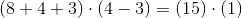We can now simply multiply these numbers together.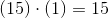Our answer is.